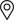Find Locally

# WHAT IS A WATT HOUR?

By Martin Camacho

As you may have noticed, we use Watt Hours to explain the capacity of most of our products. For many of us, a Watt Hour isn’t something all that familiar. So, here’s a brief explanation of what it is.

A Watt Hour is a unit of measurement for power over a period of time (an hour), or in our case, a way of measuring capacity. One Watt hour is equal to one Watt of average power flow over an hour. One Watt over four hours would be four Watt Hours of power. As an example, a 100 Watt light bulb on a 400 Watt Hour battery (like the Yeti 400) would last, on paper, 4 hours.

A Watt, the measure of power, is usually calculated using this equation: Watts = Volts x Amps. To explain a little further, we will use a plumbing analogy. If we have a water pipe; Volts would be a measure of the water pressure (force) in the pipe, Amps would be a measure of the current or flow through the pipe. A Watt would be the measure of of what you can do with that water, like turning a water wheel. So, how do we determine Watt hours?

Watt Hours are calculated using a similar equation when dealing with batteries. An example of this would be that the Yeti 400 contains a 33 Amp Hour battery operating at 12 Volts. 12 Volts x 33 Amp Hours = 396 Watt Hours or roughly 400 Wh. Not only are Watt Hours a good unit of measurement for capacity, but it is also pretty universal when finding out how many times one of our GZ products will recharge something with it’s own battery in it (like a phone, tablet, or laptop). The Equation to find the Watt Hours of a battery gives us a universal measurement despite batteries on the market varying greatly in operating voltage and mAh.